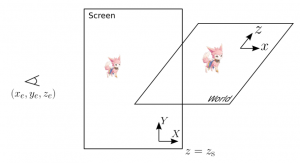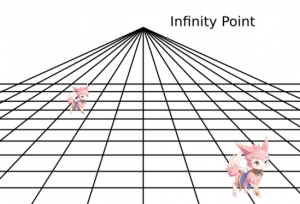# Expressing "2.5D" spaces with Cocos2d-x

## Background

These days, the terms of "dimension" is not limited to vector spaces -- in Japanese internet slang, "2.5D girls" refer to girls rendered using 3DCG. As a matter of fact, you can actually formally define dimensions that aren't integers -- fractal dimensions are in general irrational numbers.

Cocos2d-x is a game engine for 2-Dimensional games, as its name implies. However, with just a little extension, we can achieve "depth" expression -- I will introduce such an extension in this article.

The technique that we will use is "Perspective View Transformation" often used in 3DCG literature. We will scale our sprite images according to the coordinate in the depth direction, by which we achieve a virtual "depth" experience.

## ObjectiveIn this article, we consider the two coordinate systems Screen and World shown in the figure above. The Screen coordinates denote the position of the sprite on the screen, where the World coordinates denote the position of the model defined in the virtual world of our game.

We have conventionally used the cocod2d::Point class to specify the position of the sprite to be rendered. Here, we will implement its subclass PointWithDepth for our depth expression. Our objective is to implement a mapping such that by specifying the World coordinates through the PointWithDepth::SetWorldPosition method, the corresponding Screen coordinates are obtained through the cocos2d::Point (up-casted) class, and the scaling factor by the PointWithDepth::GetScale method.

## Transformation

The perspective view transformation onto the xy-plane is obtained by the following equation:

By introducing the infinite-point ( ), we can simplify the definitions of the constants:

Things that are farther away appear smaller. The scaling factor for such an effect is calculated according to:

## Implementation

Before implementing the feature in this article, we have conventionally specified the position of the character as follows:

character->setPosition(cocos2d::Point(x,y))


We can naturally extend our code for this feature by defining the following class:

class PointWithDepth:public cocos2d::Point{
...
void SetWorldPosition(float local_x,float local_y,float local_z){
m_scale=(Z_S-Z_E)/(local_z-Z_E);
cocos2d::Point::x=X_INF+m_scale*local_x;
cocos2d::Point::y=Y_INF+m_scale*local_y;
}
float GetScale(void){
return m_scale;
}
private:
float m_scale;
};


Using the PointWithDepth class, we can specify the character position and scale factor based on the World coordinates :

PointWithDepth point;
point.SetWorldPosition(local_x,local_y,local_z);
character->setPosition(point);
character->setScale(point.GetScale());


Our implementation allows us to express perspective-transformation-like effects with 2-Dimensional libraries. The figure below illustrates our output.## Conclusion

By adding just a few lines to a class that represents a point in a 2 dimensional space, we can now express our sprites in a 3 dimensional space.

You can probably apply the trial & error method and adjust some parameters to achieve the same kind of expression. However, I think we should always think systematically and mathematically before writing any kind of code -- it's the only way to assure code quality.